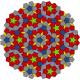# Glossary

(Will be extended from time to time.)

arc

an edge with a direction

complete graph

a complete graph is a graph, in which every vertex is connected to every other vertex

the symbol that is used for a complete graph is Kn, where n is the number of vertices

connected

a net is connected, if there is a continuous path from every vertex to every other vertex

cycle

= circuit = closed path;

a cycle is a continuous path (along edges) in which the start and end vertex are the same

cycles sum

the sum of two cycles is the set of edges that occur exactly once (meaning that the edges that occur twice will be deleted);

example:cyclomatic number

the cyclomatic number g is the number of edges that are necessary to complete a spanning tree to make this subgraph a complete connected graph

If there are v vertices and e edges in the complete connected graph, the cyclomatic number is:

g = ev +1

embedding

a graph/net, in which the vertices have been assigned (Euclidean) coordinates; this implies that now the edges have a certain length

girth

the length of the shortest cycle of a graph

if the graph is a tree, the girth is said to be infinite

graph

an abstract mathematical object in which vertices are connected by edges

k-connected

an k-connected graph is a graph in which k numbers of vertices (and their edges) have to be deleted to separate the graph into two parts

graphs, which consists of vertices which are all 3-coordinated can be indeed only 2-connected:if the two orange vertices were deleted, the graph is disjoint

minimal net

a net with genus 3

the genus of a surface is – simply speaking – equivalent to the number of its “holes” or “handles”

the primitive unit cell of a 3D-periodic net has at least 3 such holes, for most of the nets the genus is much higher

there are only 8 nets that have these minimal number of holes:
cds, dia, hms, pcu, srs, tfa, tfc, and ths

n-coordinated

an n-coordinated vertex has n connections to other vertices, n is the coordination number

shortform: n-c, for instance nbo is a 4-c net

if two or more vertices with different coordination numbers are present, the different coordination numbers of the vertices are separated by commas and are enclosed in brackets, for instance the net tbo is a (3,4)-c net

sometimes, a multinodal net is specified only in its short or compact form, i.e. only the different coordination numbers are given, in other cases every non-symmetry-related vertex is explicitely specified with its coordination number, for instance zhc is a octanodal (3,3,3,3,4,4,4,4)-c net; its short notation is again only (3,4)-c;

net

a finite or periodic, connected, and simple graph

simple means that the edges have no directions and that it contains no loops (edges linking a vertex to itself), and that there are no multiple edges between any vertices

quasiregular net

a quasiregular net is characterized by the transitivity pqrs = 1112

there is only one quasiregular net:
fcu

regular net

a regular net is characterized by the transitivity pqrs = 1111

there are only 5 regular nets:
bcu, dia, nbo, pcu, and srs

a regular net is vertex– as well as edge-transitive

semiregular net

a semiregular net is characterized by the transitivity pqrs = 11rs (with r > 1)

there are 16 semiregular nets:
lvt, sod, lcs, lcv, qtz, hxg, lcy, crs, bcs, acs, reo, thp, rhr, ana, ibb, and icc

a semiregular net is vertex– as well as edge-transitive

spanning tree

a subgraph of a graph, which is a tree and which consists of all vertices of the graph

usually a graph may have several different spanning trees

tree

a graph without any closed paths

uniform net

in a uniform net at all angles the shortest rings are all of the same size

## 5 thoughts on “Glossary”

1.Stephen hyde

1.doktorholz Post author

yes, will expand the glossary right after my holiday!

2.Stephen hyde

I was curious to remind myself of the definition… Happy holiday.

3.Thalles Diogenes

Hello,

It is a very interesting description. It could be nice to know more about MOF topology. Do you have any suggestion for literature?

Regards,

T.S. Diógenes

1.doktorholz Post author

Hi!